• 论文解读：（TransR）Learning Entity and Relation Embeddings for Knowledge Graph Completion   TransH在TransE基础上做出的改进，提高了知识表示的效果，在一定程度上解决了复杂关系的处理，同时在链接预测、...
论文解读：（TransR）Learning Entity and Relation Embeddings for Knowledge Graph Completion
TransH在TransE基础上做出的改进，提高了知识表示的效果，在一定程度上解决了复杂关系的处理，同时在链接预测、三元组分类和关系抽取任务上相比传统的方法（距离模型、非结构模型、单层神经网络、双线性模型等）达到最优，然而TransH也存在一定的问题。TransR作者发现TransH模型虽然有效的处理了复杂语义关系表示，但两个实体仍然处于相同的语义空间，因此不能够充分表示实体与关系的语义联系。
一、简要信息

序号
属性
值

1
模型名称
TransH

2
所属领域
自然语言处理

3
研究内容
知识表示

4
核心内容
knowledge embedding

5
GitHub源码
https://github.com/mrlyk423/relation_extraction 或 https://github.com/thunlp/KB2E

6
论文PDF
http://nlp.csai.tsinghua.edu.cn/~lyk/publications/aaai2015_transr.pdf)

二、摘要与引言
知识图谱的完成可以实现目标实体之间的链接预测。本文我们研究了知识图谱表征的方法。最近TransE和TransH模型通过将关系视为一种从头实体到尾实体的翻译机制来获得实体和关系的表征。事实上一个实体可能有多个不同方面（特征），关系可能关注实体不同方面的特征，公共的实体特征空间不足以表征。本文，我们提出TransR模型构建实体和关系表征，将实体空间和关系空间相分离。然后我们以这种方式训练表征向量：首先通过将实体映射到关系空间中，其次在两个投影实体之间构建翻译关系。实验中，我们在三个任务上完成了验证，分别是链接预测、三元组分类和关系抽取。实验效果表明相比之前的基线模型，包括TransE和TransR，得到了一定的提升。
完善的知识图谱旨在预测给定两个实体对的关系，即链接预测，期现如今面临的挑战包括（1）图谱中的结点包含不同类型和属性的实体、（2）边表示不同类型的关系。对于知识补全，我们不仅仅只是判断实体对是否存在关系，也需要预测具体的关系类。基于此，传统的链接预测方法则无法实现链接预测。最近一种新提出的表示学习是指将实体和关系嵌入到连续的向量空间中。
TransE和TransH模型是基于这种表示学习。TransE在word2vec的启发之下，通过构建简单的语义表示 $h+r\approx t$ 实现训练，TransH则基于TransE基础上能够对复杂关系进行表示。不过这两个模型均是假定实体和关系是在同一个语义空间中。作者提出一种新的策略，将实体和关系分别映射到不同的语义空间中，分别为entity space（实体空间）和relation space（关系空间）。
TransR的主要思路如图所示：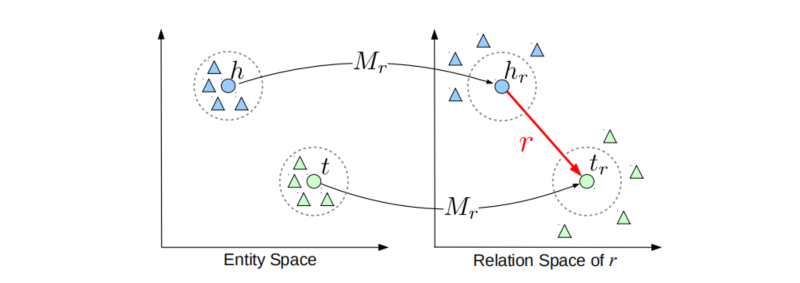假设实体对 $(h,r,t)$ ，首先根据当前的关系 $r$ 将头尾实体分别映射到关系空间中 $h_r,t_r$ ，然后在关系空间中建模 $h_r+r\approx t_r$ 。
另外，在特定的关系下，实体对通常表现出不同的模式，因此不能单纯的将关系直接与实体对进行操作。我们通过将不同的头尾实体对聚类成组，并为每个组学习不同的关系向量来扩展TransR，称为基于聚类的TransR (CTransR)。
三、相关工作与主要贡献
相关工作部分由于和TransH文章一样，请直接阅读【TransH】的相关工作部分。
四、算法模型详解（TransR）
TransR模型：设实体对 $(h,r,t)$ 的表征分别为 $\mathbf{h},\mathbf{t}\in\mathbb{R}^k$，$\mathbf{r}\in\mathbb{R}^d$，其中 $k\ne d$。对于每个关系 $r$ 给定映射矩阵 $\mathbf{M}\in\mathbb{R}^{k\times d}$ ，则有：$\mathbf{h}_r=\mathbf{h}\mathbf{M}_r$ ，$\mathbf{t}_r=\mathbf{t}\mathbf{M}_r$。得分函数定义为 $f_r(h,t)=||\mathbf{h}_r+\mathbf{r}-\mathbf{t}_r||_2^2$ 。约束条件为 $||\mathbf{h}||_2\leq1$，$||\mathbf{r}||_2\leq1$，$||\mathbf{t}||_2\leq1$，$||\mathbf{h}\mathbf{M}_r||_2\leq1$，$||\mathbf{t}\mathbf{M}_r||_2\leq1$。
CTransR模型：受到piecewise linear regression（分段线性回归）的启发。
（1）首先将输入示例分为多个组。对于特定的关系 $r$ ，所有的实体对 $(h,t)$ 可被聚类到多个簇中，每个簇中的实体对可被认为与关系 $r$ 有关。
（2）为每个簇对应的关系向量 $\mathbf{r}_c$表征，并得到对应的 $\mathbf{M}_r$ ，然后将每个簇中的头实体和尾实体映射到对应关系空间中 $\mathbf{h}_{r,c}=\mathbf{h}\mathbf{M}_r$， $\mathbf{t}_{r,c}=\mathbf{t}\mathbf{M}_r$。得分函数定义为 $f_r(h,t)=||\mathbf{h}_{r,c}+\mathbf{r}_c-\mathbf{t}_{r,c}||_2^2+\alpha ||\mathbf{r}_c-\mathbf{r}||_2^2$
TransR和CTransR的区别在于两者的关系空间不同，前者只有一个关系空间，亦即对所有的关系都在同一个空间中；后者则是根据不同的关系，对属于同一个关系的所哟实体对聚集在一个簇中，每个关系代表不同的空间。
损失函数：损失函数与之前的一样：
$L=\sum_{(h,r,t)\in S}\sum_{(h',r,t')\in S'}[\gamma + f_r(h,t)-f_r(h',t')]_+$
作者指出训练策略与负样本构建则与TransH一样，因此在此不做详细讲解。
五、实验及分析
实验主要包括三个任务，实验的具体细节与TransH相同 ，不做详细介绍。
（1）链接预测：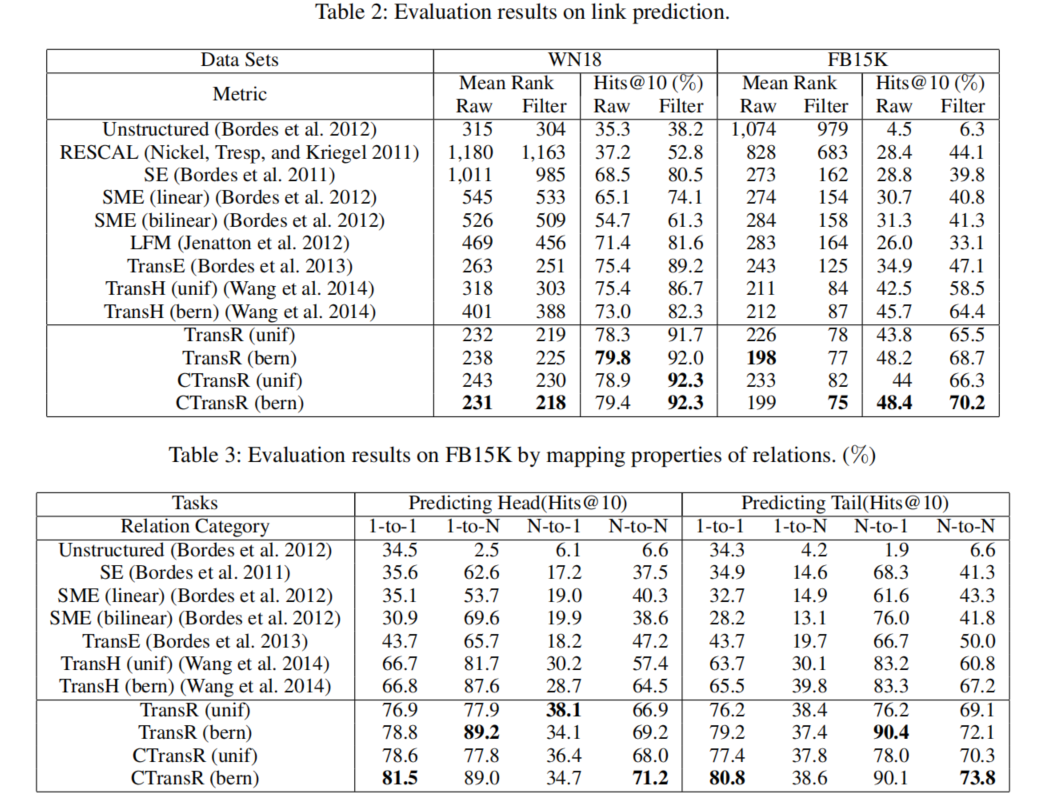（2）三元组分类：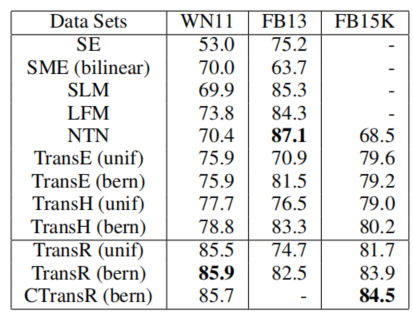（3）关系抽取：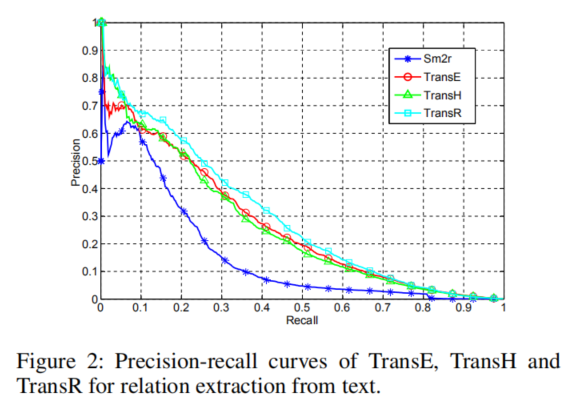六、论文总结与评价
本文作者提出的TransR和CTransR模型可以将相同关系的三元组映射到对应关系的空间中，有效的对三元组进行语义表示，在包括链接预测、三元组分类和关系抽取任务上均实现最好效果。作者提出三个未来工作，包括（1）利用推理信息增强图谱的表征；（2）探究文本与图谱的表示模型；（3）基于CTransR，研究更复杂的模型。
TransR模型巧妙的借鉴了TransH模型的空间投影想法，更细致的将不同的关系作为不同的投影空间，试想一下，每个三元组中的两个实体之所以在同一个三元组，很大程度上是因为两个实体的某些特性符合当前的关系，而这些特性在这个关系所在的语义空间中满足一定的规律，亦即 $h_r+r\approx t_r$。
TransR还有一些缺点。例如引入的空间投影策略增加了计算量、头尾实体一同投影到关系空间，而未考虑到头尾实体的不同语义类型、仅将实体投影到关系空间中还不够完全提高语义表能力等。TransD模型试图改进这些不足之处。


展开全文• TransR论文的提出还是为了来解决在TransE中无法解决的自反、一对多、多对一的关系问题。 有一个很显然的想法就是，在知识图谱中的实体和关系是不一样，那么他们存在的语义空间也就应该不一样，实体空间就是专门的来...
本文记录了阅读TransR论文时的相关内容，如果有做相关工作的同学，欢迎与我沟通联系 zhaoliang19960421@outlook.com
背景
TransR论文的提出还是为了来解决在TransE中无法解决的自反、一对多、多对一的关系问题。
有一个很显然的想法就是，在知识图谱中的实体和关系是不一样，那么他们存在的语义空间也就应该不一样，实体空间就是专门的来表示实体上的属性，关系空间就是来表示实体之间的关系内容的。
TransR就是利用这样的想法，对于实体和关系分别构建了一个空间（空间维度可以一样，也就是说当两个空间维度一样的时候实际上和TransE是一样的）。在计算三元组的势能差时，由于实体和关系不在同一个空间中，无法直接的计算，需要用一种映射方法将实体映射到对应的关系空间里面，然后利用在关系空间里面的实体的映射向量进行计算。
思路
在论文中的图像也清楚的显示TransR 和TransE的区别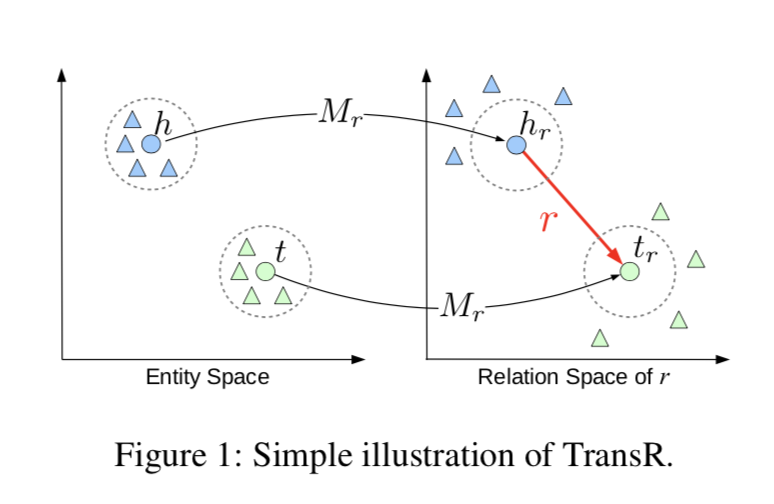对于每一个关系，都有一个映射矩阵，这个矩阵负责将实体（包括头实体和尾实体）从实体空间映射到关系空间，然后在关系空间中进行计算（论文中写的是在关系空间中进行TransE）
论文上的图片在原始的实体空间中的对于每一类实体（头实体、尾实体）中都是聚集在一起的（三角形和圆形）；但是通过映射之后，和这个关系有关的实体被聚集其它的被远离（三角形都远离了圆形，而圆形是具有这个关系的)。从图上可以看出来，通过对某个特定关系翻译矩阵的翻译功能，可以将在本来在实体空间中无法区分的实体，有了很好的区分，有了很好的区分的结果就是最终的编码效果会更好。
方法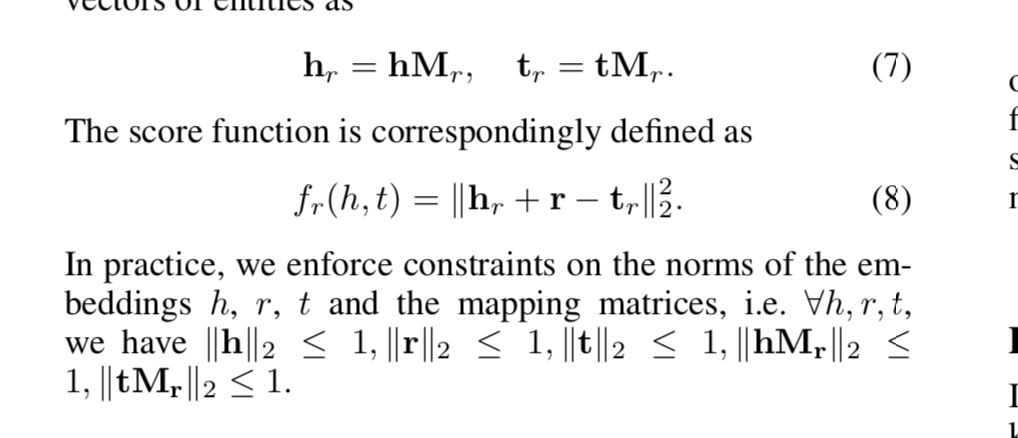具体的操作方法在原始的论文中可以看到，就是对于每个关系创建了一个映射矩阵，将实体映射到了关系空间之后就和普通的TransE一样了。
改进
在论文中又提出了一种对于TRansR的改进方法，称为CTransR，其中C是cluster聚类的意思。
具体而言，就是在进行CTransR 之前，先要进行一次初始化（论文中采用的TransE），已经得到了最初版本的向量编码结果，利用这个最初的向量编码结果来进行聚类，将同一个关系下的实体聚类到了不同的团，那么一个关系向量就被聚类成了好几个向量，不同的聚类团分别使用自己聚类团的关系向量。
那么损失函数也发生了变化，原来只是需要计算下通过特定关系的映射矩阵翻译过来的实体在关系空间中的TransE的势能差，变成了还需要计算聚类团的关系向量和标准关系向量的距离。标准关系向量也是通过初始化方法获得的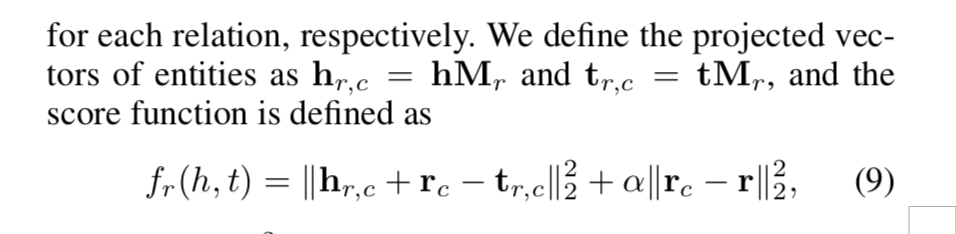论文中的$r_c$就是不同的聚类团的关系向量，$r$就是这个关系的标准向量，$M_r$就是这个关系的映射矩阵。
在CTransR中，虽然每个先将实体进行聚类之后有了不同的聚类团关系向量，但是每个关系的映射矩阵还是只有一个，这也就能说明，虽然通过聚类的方法将关系用不同的向量来表示，但是在大体上还是一样的，因为用的是一个映射矩阵，当映射矩阵都不一样了，那么就是彻底不一样了。
求助
我查看了很多网上的链接，但是都没有找到论文的原生代码，如果您恰好手中又TransR/CTransR的代码，如果可以分享的话，麻烦您通过邮件（zhaoliang19960421@outlook.com）和我联系，在此提前向您表示感谢！


展开全文• 论文链接：http://nlp.csai.tsinghua.edu.cn/~lyk/publications/aaai2015_transr.pdf 代码链接：https://github.com/mrlyk423/relation_extraction 参考文档：...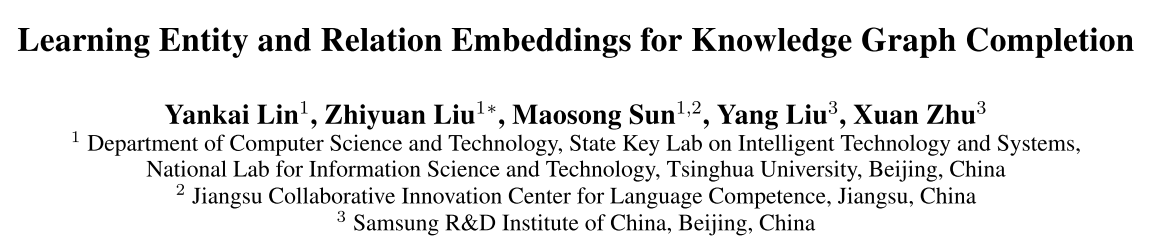论文链接：http://nlp.csai.tsinghua.edu.cn/~lyk/publications/aaai2015_transr.pdf
代码链接：https://github.com/mrlyk423/relation_extraction
参考文档：https://blog.csdn.net/qq_36426650/article/details/103357256
论文来源：2015 AAAI
导读
TransH在TransE基础上做出的改进，提高了知识表示的效果，在一定程度上解决了复杂关系的处理，同时在链接预测、三元组分类和关系抽取任务上相比传统的方法（距离模型、非结构模型、单层神经网络、双线性模型等）达到最优，然而TransH也存在一定的问题。TransR作者发现TransH模型虽然有效的处理了复杂语义关系表示，但两个实体仍然处于相同的语义空间，因此不能够充分表示实体与关系的语义联系。
1、引言
知识图谱的完成可以实现目标实体之间的链接预测。本文我们研究了知识图谱表征的方法。最近TransE和TransH模型通过将关系视为一种从头实体到尾实体的翻译机制来获得实体和关系的表征。事实上一个实体可能有多个不同方面（特征），关系可能关注实体不同方面的特征，公共的实体特征空间不足以表征。本文，我们提出TransR模型构建实体和关系表征，将实体空间和关系空间相分离。然后我们以这种方式训练表征向量：首先通过将实体映射到关系空间中，其次在两个投影实体之间构建翻译关系。实验中，我们在三个任务上完成了验证，分别是链接预测、三元组分类和关系抽取。实验效果表明相比之前的基线模型，包括TransE和TransR，得到了一定的提升。
完善的知识图谱旨在预测给定两个实体对的关系，即链接预测，期现如今面临的挑战包括（1）图谱中的结点包含不同类型和属性的实体、（2）边表示不同类型的关系。对于知识补全，我们不仅仅只是判断实体对是否存在关系，也需要预测具体的关系类。基于此，传统的链接预测方法则无法实现链接预测。最近一种新提出的表示学习是指将实体和关系嵌入到连续的向量空间中。

TransE和TransH模型是基于这种表示学习。TransE在word2vec的启发之下，通过构建简单的语义表示 $h+r\approx t$实现训练，TransH则基于TransE基础上能够对复杂关系进行表示。不过这两个模型均是假定实体和关系是在同一个语义空间中。作者提出一种新的策略，将实体和关系分别映射到不同的语义空间中，分别为entity space（实体空间）和relation space（关系空间）。

TransR的主要思路如图所示：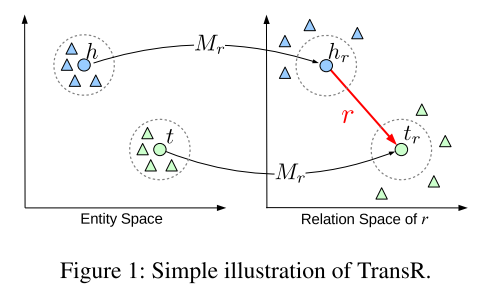假设实体对 $(h,r,t)$ ，首先根据当前的关系$r$ 将头尾实体分别映射到关系空间中 $h_r,t_r$，然后在关系空间中建模 $h_r+r\approx t_r$。

另外，在特定的关系下，实体对通常表现出不同的模式，因此不能单纯的将关系直接与实体对进行操作。我们通过将不同的头尾实体对聚类成组，并为每个组学习不同的关系向量来扩展TransR，称为基于聚类的TransR (CTransR)。
2、TransR模型详解
TransR模型：设实体对 $(h,r,t)$的表征分别为 $\mathbf{h},\mathbf{t}\in\mathbb{R}^k$，$\mathbf{r}\in\mathbb{R}^d$，其中 $k\ne d$。对于每个关系 $r$ 给定映射矩阵$\mathbf{M}\in\mathbb{R}^{k\times d}$，则有：$\mathbf{h}_r=\mathbf{h}\mathbf{M}_r$，$\mathbf{t}_r=\mathbf{t}\mathbf{M}_r$。得分函数定义为 $f_r(h,t)=||\mathbf{h}_r+\mathbf{r}-\mathbf{t}_r||_2^2$。约束条件为 $||\mathbf{h}||_2\leq1$，$||\mathbf{r}||_2\leq1$，$||\mathbf{t}||_2\leq1$，$||\mathbf{h}\mathbf{M}_r||_2\leq1$，$||\mathbf{t}\mathbf{M}_r||_2\leq1$。
CTransR模型：受到piecewise linear regression（分段线性回归）的启发。

（1）首先将输入示例分为多个组。对于特定的关系 $r$ ，所有的实体对 $(h,t)$ 可被聚类到多个簇中，每个簇中的实体对可被认为与关系 $r$ 有关。
（2）为每个簇对应的关系向量$\mathbf{r}_c$表征，并得到对应的 $\mathbf{M}_r$ ，然后将每个簇中的头实体和尾实体映射到对应关系空间中 $\mathbf{h}_{r,c}=\mathbf{h}\mathbf{M}_r$， $\mathbf{t}_{r,c}=\mathbf{t}\mathbf{M}_r$。得分函数定义为 $f_r(h,t)=||\mathbf{h}_{r,c}+\mathbf{r}_c-\mathbf{t}_{r,c}||_2^2+\alpha ||\mathbf{r}_c-\mathbf{r}||_2^2$

TransR和CTransR的区别在于两者的关系空间不同，前者只有一个关系空间，亦即对所有的关系都在同一个空间中；后者则是根据不同的关系，对属于同一个关系的所有实体对聚集在一个簇中，每个关系代表不同的空间。

损失函数：损失函数与之前的一样：$L=\sum_{(h,r,t)\in S}\sum_{(h',r,t')\in S'}[\gamma + f_r(h,t)-f_r(h',t')]_+$作者指出训练策略与负样本构建则与TransH一样，因此在此不做详细讲解。
3、实验
实验主要包括三个任务，实验的具体细节与TransH相同 ，不做详细介绍。
3.1、链接预测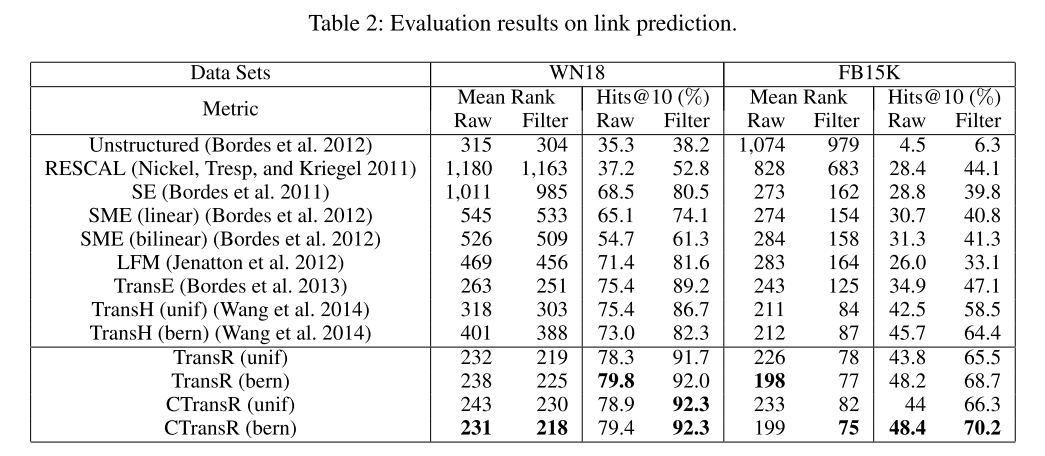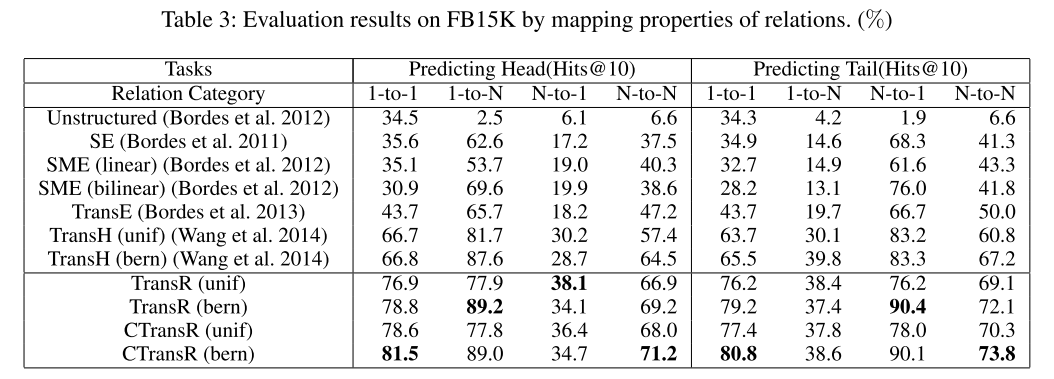3.2、三元组分类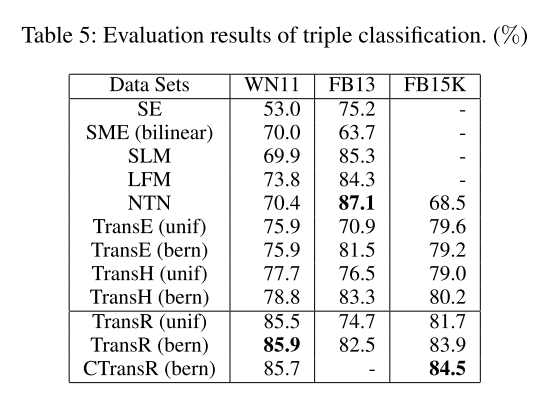3.3、关系抽取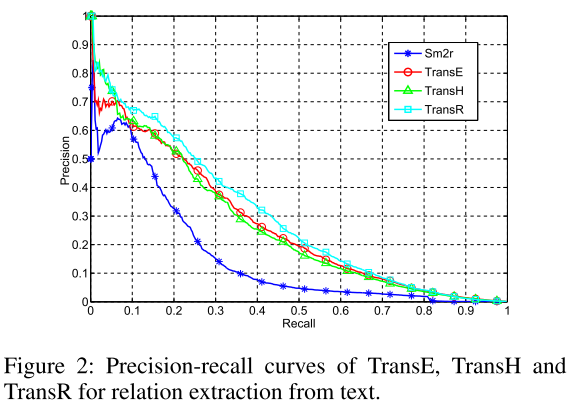4、总结
本文作者提出的TransR和CTransR模型可以将相同关系的三元组映射到对应关系的空间中，有效的对三元组进行语义表示，在包括链接预测、三元组分类和关系抽取任务上均实现最好效果。作者提出三个未来工作，包括：

（1）利用推理信息增强图谱的表征；
（2）探究文本与图谱的表示模型；
（3）基于CTransR，研究更复杂的模型。

TransR模型巧妙的借鉴了TransH模型的空间投影想法，更细致的将不同的关系作为不同的投影空间，试想一下，每个三元组中的两个实体之所以在同一个三元组，很大程度上是因为两个实体的某些特性符合当前的关系，而这些特性在这个关系所在的语义空间中满足一定的规律，亦即 $h_r+r\approx t_r$。
TransR还有一些缺点。例如引入的空间投影策略增加了计算量、头尾实体一同投影到关系空间，而未考虑到头尾实体的不同语义类型、仅将实体投影到关系空间中还不够完全提高语义表能力等。TransD模型试图改进这些不足之处。


展开全文• 最近学习了知识图谱数值表示方式的学习算法，包括TransE、TransD、TransR等，看到提到的评价指标有hit@10、mean rank等，一开始对于这些指标并不清楚。在看了一篇Transe的实现之后，明白了其所代表的含义。 表示...
最近学习了知识图谱数值表示方式的学习算法，包括TransE、TransD、TransR等，看到提到的评价指标有hit@10、mean rank等，一开始对于这些指标并不清楚。在看了一篇Transe的实现之后，明白了其所代表的含义。

表示学习的过程，其实就是实体链接的过程，首先把实体以及关系随机初始化为一定长度的向量，然后基于训练数据，希望通过类似于翻译的方式得到一个更优的向量表示，使 头实体+关系 尽可能的与尾实体的向量表示相近。在完成训练之后，如何测试、如何衡量我们得到的结果的好坏呢。

下面就是hit@10、mean rank两个指标显身手的时候了。

在测试过程中，对于一对关系及实体，我们将头实体或尾实体替换成任意一种其他的实体（共n-1个，保持另一个实体以及关系不变，只变其中一个实体），这样我们得到了（n-1）个新的关系三元组，然后我们对这些三元组计算实体关系距离，将这n-1个三元组按照距离从小到大排列。

接下来就是计算hit@10、mean rank了，在这个排好序的n-1元素中，我们从第一个开始遍历，看从第一个到第十个是否能够遇到真实的实体，如果遇到了就将（hit@10 +1），这就是hit@10的意义，表示我们的算法能够正确表示三元组关系的能力（在hit@10里  不要求第一个才是对的，能做到前十的能力就可以了）

而对于mean rank是计算在测试集里，平均到第多少个才能匹配到正确的结果。

如果还有疑惑，看了下面的代码会更清晰

        rank_head_sorted = sorted(rank_head_dict.items(),key = operator.itemgetter(1))
rank_tail_sorted = sorted(rank_tail_dict.items(),key = operator.itemgetter(1))

#rank_sum and hits
if i<10:
hits += 1 #如果不到十次就命中了
rank_sum = rank_sum + i + 1 #统计总命中次数，mean rank就是对这个总数计算平均值
break

for i in range(len(rank_tail_sorted)):
if triple == rank_tail_sorted[i]:
if i<10:
hits += 1
rank_sum = rank_sum + i + 1
break
step += 1
if step % 5000 == 0:
print("step ", step, " ,hits ",hits," ,rank_sum ",rank_sum)
print()

self.hits10 = hits / (2*len(self.test_triple))  #这个是计算的前十个命中的概率
self.mean_rank = rank_sum / (2*len(self.test_triple)) #这个是计算平均第几个命中


展开全文知识图谱
• 摘要： 知识图谱补全以执行实体间的链接预测为目标。本文，我们考虑知识图谱嵌入方法。近年来，一些模型像TransE和TransH通过把关系作为从头实体到尾实体的翻译来建立...本文，我们提出了TransR，分别在实体空间和关
• ## 对transR的理解

千次阅读 2018-09-16 17:05:56
• 该项目将不再维护，建议用户访问和使用新软件包 。
• r,t映射到同一个空间, TransR将r独立出来, 即对于每个关系 rir_iri​, 有一个映射矩阵 MriM_{r_i}Mri​​, 得 h⃗=Mrih\vec{h}=M_{r_i}hh =Mri​​h, t⃗=Mrit\vec{t}=M_{r_i}tt =Mri​​t. 如果 h⃗−t⃗⃗\vec{h}-...
• TransR通过关系矩阵将实体映射到关系空间，再最小化三元组的距离。 其中， h r = h M r h_{r}=hM_{r} h r ​ = h M r ​ ， t r = t M r t_{r}=tM_{r} t r ​ = t M r ​ 。 3.应用 推荐系统 Relational...知识图谱
• 1.TransR: 与transH基本类似，这里不再赘述 2.CTransR： 文中用(h-t)的差值作为聚类的参数，h和t的值通过transE模型计算得出，聚类之后，对每一类学习一个rc向量和Mr向量，代表关系中的一个子模式，所以score ...
• 知识表示学习的基本思想就是把知识图谱中的三元组...TransR 认为实体空间和关系空间应该是不同的。 实体 h 和 t 映射到关系空间中再做这种平移变换。 对于每一个关系有一个与之对应的r,和 M r M_r M r ​ 。TransE TransH
• 因此，TransR模型的损失函数为: f r ( h , t ) = ∣ ∣ h r + r − t r ∣ ∣ l 1 , l 2 f_r(h,t)=||h_r + r - t_r||_{l_1,l2} fr​(h,t)=∣∣hr​+r−tr​∣∣l1​,l2​ TransR缺点 模型利用了复杂的矩阵计算，将...
• TransR 标题: Learning Entity and Relation Embeddings for Knowledge Graph Completion（2015） TransE和TransH模型都假设实体和关系是语义空间中的向量，因此相似的实体在同一实体空间中会非常接近。 然而，每个...
• 整理转自：fffnull 的 CSDN 博客，感谢他的翻译分享 论文原文地址：Learning Entity and Relation Embeddings for Knowledge Graph Completion论文翻译
• 基于PyTorch的知识图谱表示，实现了TransE、TransH、TransR、TransD四种算法知识图谱 知识表示
• TransE无法解决的自反、一对多、多对一关系问题，在TransR/CTransR中通过将实体和关系在不同空间表示的方法进行了解决。 TransR的做法是让实体空间单纯的表示实体的属性信息，关系空间单纯的表示实体之间的关系，...
• KG Embedding学习，只涉及TransE、TransH、TransR、TransDNLP TransE
• 论文解读：（TransD）Knowledge Graph ...先前提出的TransE、TransH、TransR模型对表示学习提升不少，表示学习对关系抽取、三元组分类以及链接预测等方面具有作用。TransD模型改进TransR，认为不同的实体应映射到...
• 在本教程中，我将全面介绍知识图谱推理的最新进展，包括:(1)知识图谱嵌入的方法(如TransE、TransR和RotatE);(2)传统的归纳逻辑规划方法和最新的神经逻辑规划方法;(3)结合神经和符号逻辑方法进行知识图谱推理的最新...
• ## 数据集Freebase-FB13

千次阅读 2019-12-13 09:09:09
数据集： FB13是Freebase的子集，包含13种关系和75k种实体。 相关论文有TransE、TransH、TransR、TransD以及KG2E。
• 数据集：WN11是WordNet的子集，包含11种关系和38k种实体。 相关论文有TransE、TransH、TransR、TransD以及KG2E。
• 来自：AI自然语言处理与知识图谱知识表示学习Trans系列梳理导语：本文将简短梳理知识表示学习Trans系列方法，包含TransE、TransH、TransR、TransD、TransA......